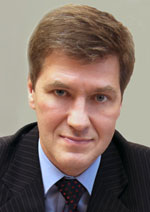Publications
Editor-in-ChiefNikiforov
D.Sc., Prof.
Partners

DOI: 10.17586/2226-1494-2016-16-1-174-180

# WENO SCHEMES FOR SOLUTION OF UNSTEADY ONE-DIMENSIONAL GAS DYNAMICS TEST PROBLEMS

P. V. Bulat, K. N. Volkov

Read the full article';
Article in Russian

For citation: Bulat P.V., Volkov K.N. WENO schemes for solution of unsteady one-dimensional gas dynamics test problems. Scientific and Technical Journal of Information Technologies, Mechanics and Optics, 2016, vol. 16, no. 1, pp. 174–180.

Abstract

Creation of test solutions is an essential element in the general design contents for numerical methods aimed at integration of Euler equations. We consider numerical solution of Euler equations describing flows of inviscid compressible gas and allowing continuous and discontinuous solutions. Discretization of Euler equations is based on finite volume method and WENO finite difference schemes. The numerical solutions computed are compared with the exact solutions of Riemann problem. Monotonic correction of derivatives makes it possible to avoid new extremes and ensures monotonicity of the numerical solution near the discontinuity, but it leads to the smoothness of the existing minimums and maximums and to the loss of accuracy. Calculations with the use of WENO schemes allow obtaining accurate and monotonic solution with the presence of both weak and strong gas dynamical discontinuities.

Keywords: gas dynamics, finite difference scheme, shock wave, rarefaction wave, contact discontinuity, Riemann problem

Acknowledgements. The study has been carried out under financial support of the Ministry of Education and Science of the Russian Federation (agreement No.14.575.21.0057).

References

1. Toro E.F. Riemann Solvers and Numerical Methods for Fluid Dynamics. Berlin, Springer-Verlag, 2009, 724 p. doi: 10.1007/b79761
2. Kulikovskii A.G., Pogorelov N.V., Semenov A.Yu. Matematicheskie Voprosy Chislennogo Resheniya Giperbolicheskikh Sistem Uravnenii [Mathematical Problems in the Numerical Solution of Hyperbolic Systems]. Moscow, Fizmatlit Publ., 2001, 608 p.
3. Wesseling P. Principles of Computational Fluid Dynamics. Springer, 2000, 664 p. doi: 10.1007/978-3-642-05146-3
4. Volkov K.N., Deryugin Yu.N., Emel'yanov V.N., Kozelkov A.S., Teterina I.V. Raznostnye Skhemy v Zadachakh Gazovoi Dinamiki na Nestrukturirovannykh Setkakh [Difference Schemes in Gas Dynamic Problems on Unstructured Grids]. Moscow, Fizmatlit Publ., 2014, 412 p.
5. Bulat P.V., Volkov K.N. Use of WENO schemes for simulation of the reflected shock wave–boundary layer interaction. Journal of Engineering Physics and Thermophysics, 2015, vol. 88, no. 5, pp. 1203–1209. doi: 10.1007/s10891-015-1301-8
6. Bulat P.V., Volkov K.N. Simulation of supersonic flow in a channel with a step on nonstructured meshes with the use of the WENO scheme. Journal of Engineering Physics and Thermophysics, 2015. doi: 10.1007/s10891-015-1346-8
7. Kermani M.J., Plett E.G. Modified entropy correction formula for the Roe scheme. Proc. 39th Aerospace Sciences Meeting and Exhibit. Reno, USA, 2001.
8. Pandolfi M., D'Ambrosio D. Numerical instabilities in upwind methods: analysis and cures for the carbuncle phenomenon. Journal of Computational Physics, 2001, vol. 166, no. 2, pp. 271–301. doi: 10.1006/jcph.2000.6652
9. Svetsov V. Vortical regime of the flow behind the bow shock wave. Shock Waves, 2001, vol. 11, no. 3, pp. 229–244.
10. Chauvat Y., Moschetta J.-M., Gressier J. Shock wave numerical structure and the carbuncle phenomenon. International Journal for Numerical Methods in Fluids, 2005, vol. 47, no. 8–9, pp. 903–909. doi: 10.1002/fld.916
11. Quirk J.J. Contributed to the great Riemann solver debate. International Journal for Numerical Methods in Fluids, 1994, vol. 18, no. 6, pp. 555–574.
12. Liska R., Wendroff B. Comparison of several difference schemes on 1D and 2D test problems for the Euler equations. SIAM Journal of Scientific Computing, 2003, vol. 25, no. 3, pp. 995–1017. doi: 10.1137/S1064827502402120
13. Mandal J.C., Panwar V. Robust HLL-type Riemann solver capable of resolving contact discontinuity. Computers and Fluids, 2012, vol. 63, pp. 148–164. doi: 10.1016/j.compfluid.2012.04.005
14. Elizarova T.G., Shil'nikov E.V. Capabilities of a quasi-gasdynamic algorithm as applied to inviscid gas flow simulation. Computational Mathematics and Mathematical Physics, 2009, vol. 49, no. 3, pp. 532–548. doi: 10.1134/S0965542509030142
15. Xiong T., Shu C.-W., Zhang M. WENO scheme with subcell resolution for computing nonconservative Euler equations with applications to one-dimensional compressible two-medium flows. Journal of Scientific Computing, 2012, vol. 53, no. 1, pp. 222–247. doi: 10.1007/s10915-012-9578-7
16. Woodward P.R., Colella P. The numerical simulation of two-dimensional fluid flow with strong shocks. Journal of Computational Physics, 1984, vol. 54, no. 1, pp. 115–173. doi: 10.1016/0021-9991(84)90142-6
17. Hannappel R., Hauser T., Friedrich R. A comparison of ENO and TVD schemes for the computation of shock-turbulence interaction. Journal of Computational Physics, 1995, vol. 121, no. 1, pp. 176–184. doi: 10.1006/jcph.1995.1187
18. Shu C.-W., Osher S. Efficient implementation of essentially non-oscillatory shock-capturing schemes II. Journal of Computational Physics, 1989, vol. 83, no. 1, pp. 32–78. doi: 10.1016/0021-9991(89)90222-2
19. Breslavskiy P.V., Mazhukin V.I. Modeling of shock waves interaction on dynamically adapting grids. Matematicheskoe Modelirovanie, 2007, vol. 19, no. 11, pp. 83–95.
20. Kudryavtsev A.N., Poplavskaya T.V., Khotyanovsky D.V. Application of high-order accuracy schemes to numerical simulation of unsteady supersonic flows. Matematicheskoe Modelirovanie, 2007, vol. 19, no. 7, pp. 39–55.